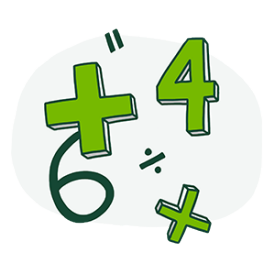### >

Probability allows us to predict how often an outcome or win will occur, but does not allow us to predict when exactly it will happen. It’s useful to know the difference in order to make informed decisions about the games you choose to play and to consider as a game strategy if applicable.

### >In gambling, the concept of odds is different for table games and slot machines. For table games, odds refer to the total amount you are paid if your bet wins, and is typically expressed in ratios.  Odds can also be referred to as a ‘payout’.  For example, if the payout is 2 to 1 (2:1), this means that for each \$1 wager you make, any winning outcome will pay you a total of \$2.

Understanding the difference between the odds and probability helps us determine the house advantage. The bigger the difference between the odds and probability, the bigger the house advantage.

##### An example with roulette

Let’s say you’re playing roulette, and you place a \$10 bet that a red number will come up next.
The odds state that the bet pays 1 to 1. If you win, your \$10 bet would pay \$10.

But the probability of the ball landing on a red number is 47.4 percent. We calculate the probability like this:

• There are 18 red numbers on the roulette wheel.
• And 38 total numbers that could come up: 18 red, 18 black, 0 and 00.
• 18 / 38 = 47.37%.

Over time, you would expect to win 47.4 percent of the time. But you’re being paid as if the probability was 50 percent. The difference between the two helps us determine the house advantage.

The odds for a game are typically listed somewhere close by. In craps, for example, they’re usually written right on the craps table.

### >

For the lottery, odds and probability mean the same thing. They both refer to the mathematical likelihood of winning. For example, the odds of winning a 6/49 jackpot are 1 in 13,983,816. Expressed as a probability, you have a 0.00000715112384 percent chance of winning. In other words, it’s very unlikely.